# How to Use SUMPRODUCT in Excel and Google Sheets

SUMPRODUCT is a useful function in both Excel and Google Sheets for calculating total prices. Check out this tutorial for using the function in both softwares.1. How SUMPRODUCT works
2. Use SUMPRODUCT to two columns
3. Use SUMPRODUCT to three or more columns
4. How to add only the entries of the same item in SUMPRODUCT
5. References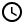5 Minutes

One useful function for calculating total prices is the SUMPRODUCT function. Both Excel and Google Sheets have this function. In this tutorial, we will learn how to use SUMPRODUCT in both Excel and Google Sheets.

## How SUMPRODUCT works

The SUMPRODUCT multiplies the numbers along the same row, and then adds the products to arrive at the answer. To make it clearer, we added an illustration below:

We are given two columns A and B with three cells each. What SUMPRODUCT will do is to multiply A1 to B1, A2 to B2, and A3 to B3. Then, SUMPRODUCT will add these products to get the final answer. The answer it gives is A1*B1 + A2*B2 + A3*B3.

The SUMPRODUCT function has the following syntax:

=SUMPRODUCT(Column1,Column2)

Where Column1 and Column2 are the ranges of selected columns 1 and 2. The two columns must have the same length.

In the next examples we will see how it works in both Excel and Google Sheets.

## How to use SUMPRODUCT with two columns

Let us start with a simple example. We want to get the total price of the items bought from your store: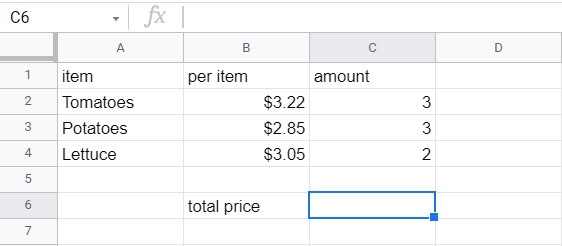Items brought from your store. Placed in Google Sheets. The same data is used in Excel.

As you can see, you can easily apply the SUMPRODUCT function here. Simply specify the two rows as B2:B4 and C2:C4:

=SUMPRODUCT(B2:B4,C2:C4)

The result is: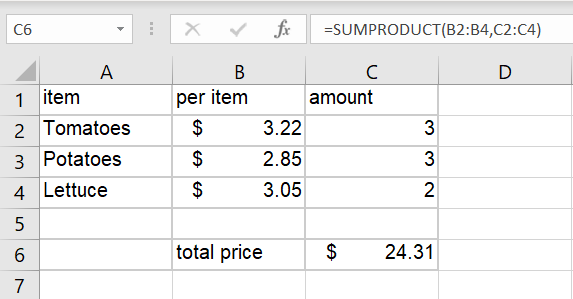SUMPRODUCT for two columns in Excel.

As you can see, SUMPRODUCT is a wonderful function that you can use to quickly calculate the total price of the items bought, as long as each column is the same length.

## How to use SUMPRODUCT with three or more columns

SUMPRODUCT can also be used to multiply and sum three or more columns together. The formula will multiply the three numbers along the same row together and then find the sum of the products. For example, we have the following data:

We simply apply SUMPRODUCT to these three columns:

=SUMPRODUCT(A1:A7,B1:B7,C1:C7)

The result is:

## How to add specific rows with a specific value with SUMPRODUCT

The SUMPRODUCT allows you to specify which items to add on the list. For example, we want to add only the Lettuce items in the following list: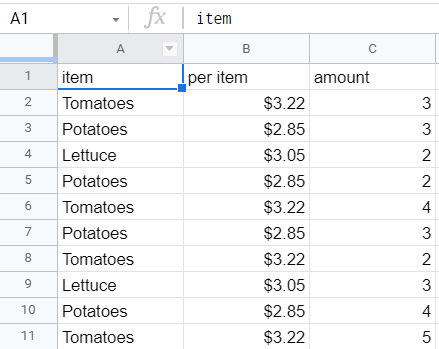A long list of products with their price per item and the amount of those products bought.

There is a way to do so. You add a multiplier that gives a value of 1 when it is equal to a certain value and a value of 0 if it is not equal. The multiplier turns out to be a simple function:

=(A2=\$A\$13)

In this example, the cell A13 contains the word or value  with which we want to compare the values in Column A. This will give a TRUE or FALSE value: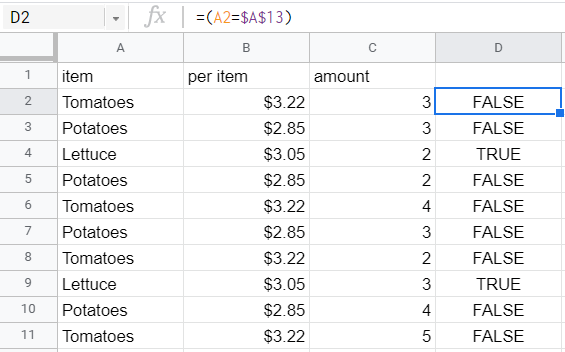The given equation above applied to the entire column. The result is in the last column.

We will then add this to our SUMPRODUCT function. If we want to add all the prices, we will have the following function:

=SUMPRODUCT(B2:B11,C2:C11)

However, since we want to limit those added to the items listed in A13, we now have the following function:

=SUMPRODUCT((A2:A11=A13)*B2:B11,C2:C11)

The result is: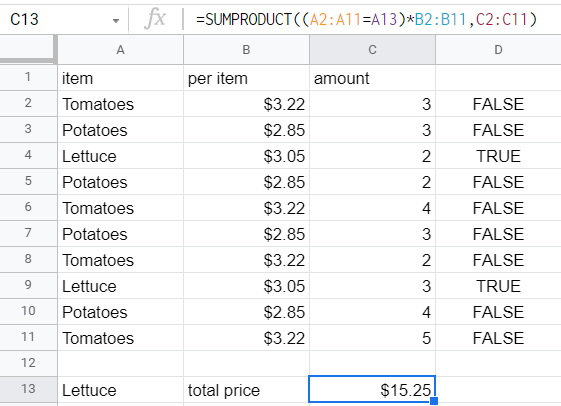The SUMPRODUCT plus special conditional function applied to the list in Google Sheets.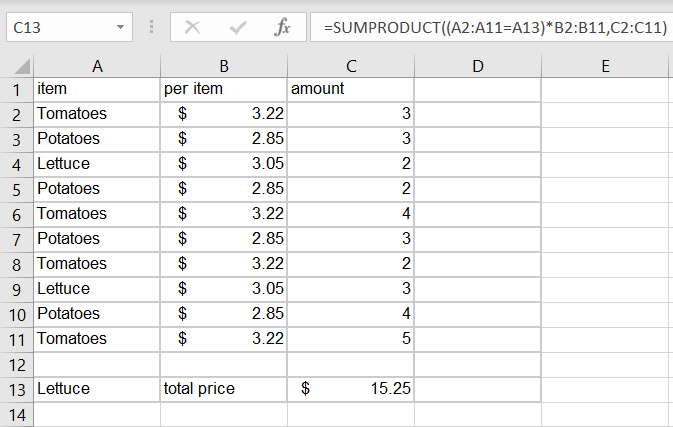The SUMPRODUCT plus special conditional function applied to the list in Excel.

## References

SUMPRODUCT - Docs Editors Help

SUMPRODUCT function - Office Support

#### Enter Your Email Below to Try Lido for FREE!

Experience all of the benefits of the classic spreadsheet with much more powerful features that save users time!
• One Click Data Imports From Anywhere
• Transform Spreadsheets into Software in Seconds
• Pre-built & Custom Templates
• Visualize Real-Time Data in Centralized Dashboards
100% FREE - No Credit Card Required